## Monday, April 29, 2019

### (x, why?) Mini: iStruggle

(Click on the comic if you can't see the full image.)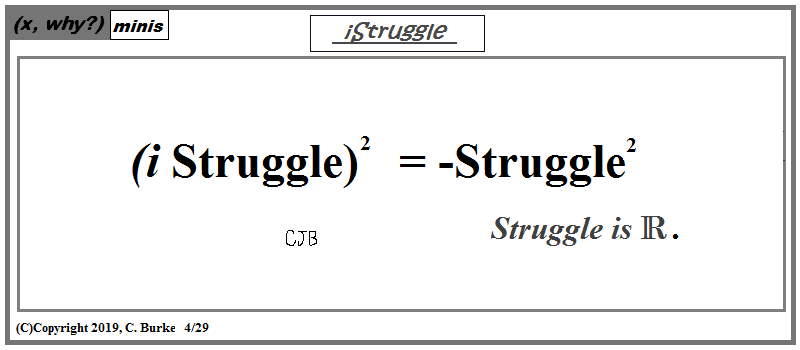iStruggle is imaginary or a trademark of a certain corporation ...

Come back often for more funny math and geeky comics.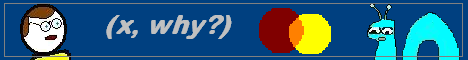## Sunday, April 28, 2019

### Numerology

(Click on the comic if you can't see the full image.)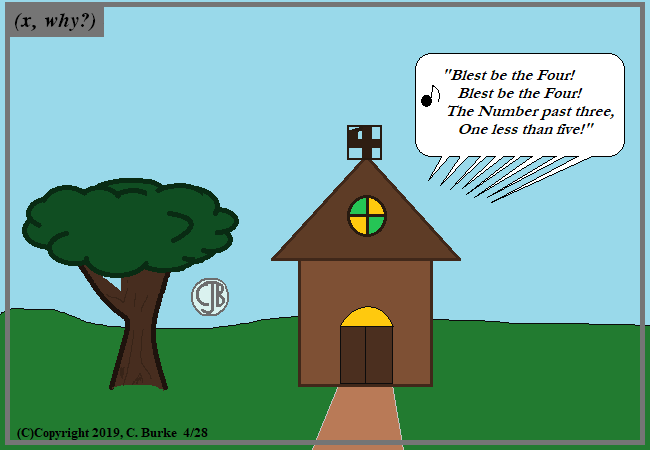LIVE in the IV!
The opposite is the VI, which is EVIL.

Four is the one TRUE number because it has 4 letters in its proper name. However, La Iglesia del Cinco might disagree.

Writer's notes: I almost went with "Number Theology" for a title, even if that was on the comic itself, but not the heading on the blog post.

Also, I'm thankful that "Less is More", and not just becaue four is a small number, but because I would've agonized for days over the rest of the verse. Something like:

... I will not fear / the odd or prime / or the fractions that would divide!
... which sort of rhymes with "five". Also "number line" could've been worked in there.

Come back often for more funny math and geeky comics.## Tuesday, April 23, 2019

### (x, why?) Mini: Morrie's Law

(Click on the comic if you can't see the full image.)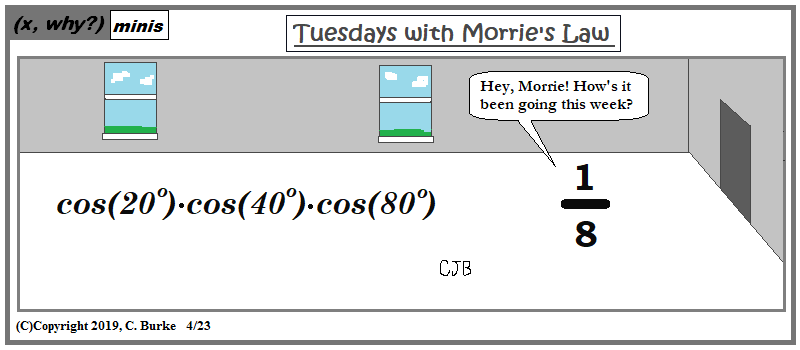He's a Fine Man for visiting every week!

Come back often for more funny math and geeky comics.## Sunday, April 21, 2019

### Happy Easter 2019!

(Click on the comic if you can't see the full image.)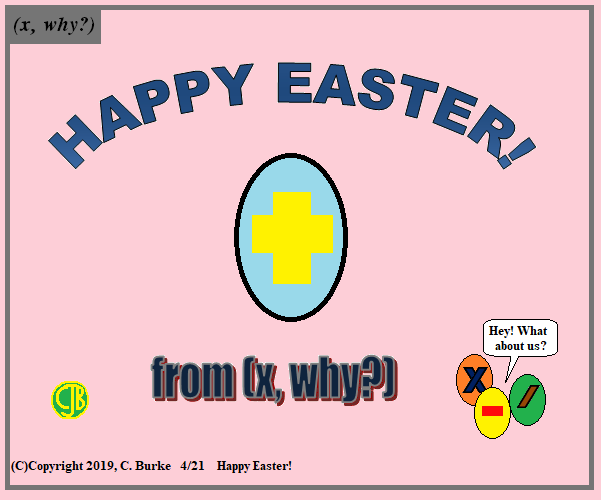He is Risen, so that's a Big Plus!

Come back often for more funny math and geeky comics.## Thursday, April 18, 2019

### Endgame

(Click on the comic if you can't see the full image.)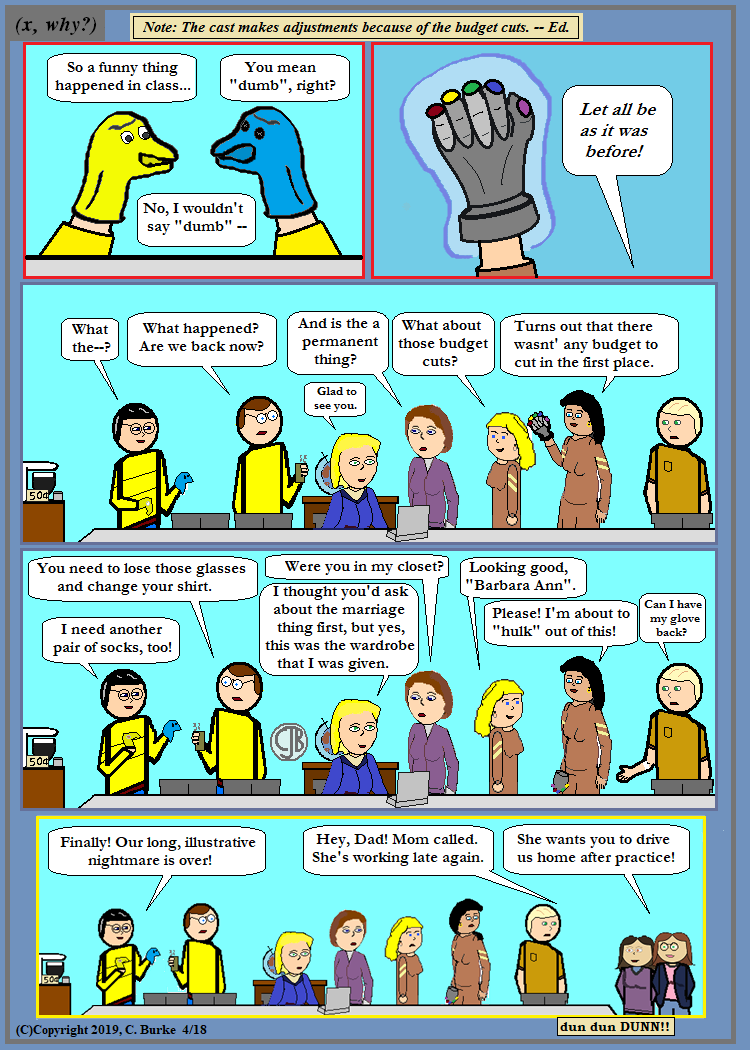Is everything back to normal? Maybe those two are just colluding ... but we haven't found any evidence of that yet.

I hope you enjoyed this silliness as much as I enjoyed perpetrating it!

Come back often for more funny math and geeky comics.## Friday, April 12, 2019

### Tryouts

(Click on the comic if you can't see the full image.)Names? I'm getting there.

Four more students got speaking roles. And those two from the other school showed up.

Tidbit about those two transfer students: they were based on some students I met while teaching at my first new school in a decade, and when Mike was asked to leave his school. Those two appear in several of the comics that wound up in the Logic As a Tool textbook. My "regular" students did not.

Come back often for more funny math and geeky comics.## Wednesday, April 10, 2019

### January 2019 Common Core Geometry Regents, Part I (multiple choice)

The following are some of the multiple questions from the recent January 2019 New York State Common Core Geometry Regents exam.
The answers to Part II can be found here
The answers to Parts III and IV can be found here

### January 2019 Geometry, Part I

Each correct answer is worth up to 2 credits. No partial credit. Work need not be shown.

1. After a dilation with center (0,0), the image of DB is D'B'. If DB = 4.5 and D'B' = 18, the scale factor of this dilation is

18 / 4.5 = 4. D'B' is four times bigger than DB.

2. In the diagram below, triangle ABC with sides of 13, 15, and 16, is mapped onto triangle DEF after a clockwise rotation of 90° about point P.

If DE = 2x - 1, what is the value of x?

In a rotation, size is preserved. Side AB maps on side DE, so 2x = 1 = 16
2x = 17
x = 8.5

3. On the set of axes below, triangle ABC has vertices at A(2,0), B(2,4), C(4,2), and triangle DEF has vertices at D(4,0), E(4,8), F(8,4).

Which sequence of transformations will map ABC onto DEF?

Answer: (3) a dilation of ABC by a scale factor of 2 centered at the origin, followed by a rotation of 180° about the origin
ABC is the smaller triangle. To map it onto DEF, you need a scale factor of 2, not 1/2, which eliminates (2) and (4). However, just doubling the size will not adjust the orientation, so (1) is out.

4. The figure below shows a rhombus with noncongruent diagonals.

Which transformation would not carry this rhombus onto itself?

Answer: (3) a clockwise rotation of 90° about the intersection of the diagonals
If you rotate 90 degrees, the horizontal lines become vertical lines. The rhombus doesn't have vertical lines, so it isn't going to map onto itself.

5. In the diagram below of circle O, points K, A, T, I, and E are on the circle, triangle KAE and triangle ITE are drawn, arc KE = arc EI, and ∠EKA = ∠EIT.

Which statement about triangle KAE and triangle ITE is always true?

Arc KE = arc EI gives us two pieces of information: Chords KE and EI are congruent, and ∠A and ∠T are congruent. We are given ∠EKA = ∠EIT. By AAS, the triangles are congruent. We have no information that they are right triangles (they could be), but this choice can logically be eliminated. Even if they were right triangles, one of the other three choices would have to be true!

6. In right triangle ABC shown below, point D is on AB and point E is on CB such that AC || DE.

If AB = 15, BC = 12, and EC = 7, what is the length of BD?

The triangles are proportional in size, but we need to know the length of side BE.
If EC = 7, then BE = 12 - 7 = 5.
AB / BC = BD / BE
15 / 12 = BD / 5
12 BD = (15)(5)
12 BD = 75
BD = 6.25

7. In rhombus VENU, diagonals VN and EU intersect at S. If VN = 12 and EU = 16, what is the perimeter of the rhombus?

If the diagonals have lengths 12 and 16 and they bisect each other, then the rhombus can be divided into four right triangles with legs of 6 and 8. That makes the hypotenuses of those triangles equal to 10, which is the length of one side of the rhombus. So the perimeter is 40.

8. Given right triangle ABC with a right angle at C, m∠B = 61°. Given right triangle RST with a right angle at T, m∠R = 29°.

Which proportion in relation to triangle ABC and triangle RST is not correct?

Angle A = Angle R, Angle B = Angle S. AB corresponds to RS, BC corresponds to ST, and AC corresponds to RT.
In Choice (1), the second ratio is upside down. It goes big to small equals small to big.

9. A vendor is using an 8-ft by 8-ft tent for a craft fair. The legs of the tent are 9 ft tall and the top forms a square pyramid with a height of 3 ft.

What is the volume, in cubic feet, of space the tent occupies?

The bottom of the tent is a prism. L * W * H = 8 * 8 * 9 = 576 (Eliminate Choice (1).)
The top of the tent is a prism. 1/3 * L * W * H = 1/3 * 8 * 8 * 3 = 64.
Total is 640.

10. In the diagram below of right triangle KMI, altitude IG is drawn to hypotenuse KM.

If KG = 9 and IG = 12, the length of IM is

Hopefully, you realized right away that triangle IGK is a Pythagorean Triple, 9-12-15, a multiple of the 3-4-5 right triangle.
Also triangles IGK, IGM and IKM are all similar, so each of them is proportional and a multiple of 3-4-5.
IK is the hypotenuse of the IGK, and the shorter leg of IKM. Triangle IKM is 15-4n-5n. Since 15 = 3 times 5, the other two legs are 4 * 5 = 20 and 5 * 5 = 25. So IM is 20.

The other way to do this: The Right Triangle Altitude Theorem tells us that KG/IG = IG/GM.
So (KG)(GM) = (IG)2
(9)(GM) = (12)2
(9)(GM) = 144
GM = 16.
IG = 12, GM = 16. By Pythagorean Theorem (or recognizing 3-4-5), IM = 20.

11. Which three-dimensional figure will result when a rectangle 6 inches long and 5 inches wide is continuously rotated about the longer side?

Answer: (3) a cylinder with a radius of 5 inches and a height of 6 inches
Rotating a rectangle about an axis will result in a cylinder because the rectangle is traveling a circular path. Eliminate (1) and (2).
If it's rotating about the longer side than that side will be the height. The side moving is a circle will be the radius.

12. Which statement about parallelograms is always true?

Answer: (2) The diagonals bisect each other.
This is true for all parallelograms. Choice (1) is only true for rectangles. Choice (3) is only true for rhombuses. Choice (4) is only true for squares.

13. From a point on the ground one-half mile from the base of a historic monument, the angle of elevation to its top is 11.87°. To the nearest foot, what is the height of the monument?

One-half mile is (1/2)(5280) = 2640 feet
You have adjacent (the distance) and you need the opposite (the height). So use Tangent.
tan 11.87° = x / 2640
x = 2640 tan 11.87° = 554.89...

14. 4 The area of a sector of a circle with a radius measuring 15 cm is 75π cm2. What is the measure of the central angle that forms the sector?

A = π r2 = π(15)2 = 225π.
225π / 75π = 360 / x. (At this point, drop the π, because they factor out.)
225x = 75 * 360
225x = 27000
x = 120

15. 5 Point M divides AB so that AM:MB = 1:2. If A has coordinates (-1,-3) and B has coordinates (8,9), the coordinates of M are

The ratio 1:2 means that point M is 1/3 of the distance away from point A and 2/3 of the distance from point B.
Find 1/3 of the difference of the x-coordinates and 1/3 of the difference of the y-coordinates.
(8 - -1)/3 = 9/3 = 3. -1 + 3 = 2, so the x-coordinate of M is 2. The answer must be Choice (1).
(9 - -3)/3 = 12/3 = 4. -3 + 4 = 1, so the y-coordinate of M is 1.

16. In the diagram below of triangle ABC, AC is extended through point C to point D, and BE is drawn to AC.

Which equation is always true?

Answer: (4) m∠7 = m∠3 + m∠2

The Exterior Angle Theorem states that the exterior angle of a triangle equals the sum of the two remote angles. This is because ∠2 + ∠3 + ∠4 = 180, because a triangle has 180 degrees, and ∠7 + ∠4 = 180, because supplementary angles add up to 180 degrees. So ∠2 + ∠3 + ∠4 = ∠7 + ∠4 and therefore ∠2 + ∠3 = ∠7.

17. In the diagram below of right triangle ABC, AC = 8, and AB = 17.

Which equation would determine the value of angle A?

Answer: (4) tan A = 15/8
You have the adjacent side and the hypotenuse, so the easiest way to find A would be cos A = 8/17. However, that isn't one of the choices, so we need to find something else.
Notice that 15 is in the choices. Where does this come from? It's the missing leg of the triangle.
Again, this is a common Pythagorean Triple: 8-15-17. However, if you didn't notice this, you can figure it out:
172 - 82 = 289 - 64 = 225 = 152
Now look at the choices:
Choice (2) has the adjacent over the opposite, instead of the other way around.
Choice (4) has opposite over adjacent, which is correct.

18. Francisco needs the three pieces of glass shown below to complete a stained glass window. The shapes, two triangles and a trapezoid, are measured in inches.

Glass can be purchased in rectangular sheets that are 12 inches wide. What is the minimum length of a sheet of glass, in inches, that Francisco must purchase in order to have enough to complete the window?

The two smaller triangles can be placed adjacent to the trapezoid, matching up the side lengths, to create a rectangle that is 12 x 20 inches.

19. In the diagram of quadrilateral NAVY below, m∠YNA = 30°, m∠YAN = 38°, m∠AVY = 94°, and m∠VAY = 46

Which segment has the shortest length?

Angle V is 94 and angle VAY = 46, so angle AYV = 180 - (94 + 46) = 30. The smallest side of triangle VAY is the short across from the shortest angle, which would be VA.

20. What is an equation of a circle whose center is (1,4) and diameter is 10?

Answer: (1) x2 - 2x + y2 - 8y = 8
(x - h)2 + (y - k)2 = r2
(x - 1)2 + (y - 4)2 = 52 (Diameter is 10. Radius is 5.)
x2 - 2x + 1 + y2 - 8y + 16 = 25 (Eliminate Choices (2) and (4).)
x2 - 2x + y2 - 8y = 25 - 16 - 1 = 8 Choice (1)

21. On the set of axes below, ABC, altitude , and median are drawn.

Which expression represents the area of triangle ABC?

The area of a triangle is 1/2 times base times altitude. Notice I said "altitude" and not "height". To get an altitude, we need a right angle. You can tell a right angle on a graph by perpendicular lines, which have negative reciprocal slopes.
MC has a slope of -1. None of the sides have a slope of 1.
GC has a slope of -1/2. Side AB has a slope of 2, which is the negative reciprocal. So AB is the base and GC is an altitude.

22. In right triangle ABC, m∠C = 90° and AC != BC. Which trigonometric ratio is equivalent to sin B?

Since AC is not equal to BC, the angles cannot be 45 degrees each, which would mean there were two possible answers.
Sin B is always the same as cos A.
This is because the side opposite B is adjacent to A.

23. As shown in the diagram below, the radius of a cone is 2.5 cm and its slant height is 6.5 cm.

How many cubic centimeters are in the volume of the cone?

You're given the slant height, but you need the height. The slant height forms a right triangle with the height and the radius.
So h = (6.52 - 2.52)(1/2) = 6.
Hint: if you look at the answers, not of the multiples of pi are irrational, meaning that the sides of the right triangle form a Pythagorean Triple. If you double the numbers given to get rid of the decimals, we have 5/?/13. There's a 5-12-13 right triangle. Half of 12 is 6.
If you didn't see that, you still have Pythagorean Theorem and a calculator.

Volume of a cone is 1/3 * r2 * h * π = (1/3)(2.5)2(6) * π = 12.5π

Common mistakes: using the slant height as the height, and forgetting the (1/3). Both of those are incorrect choices.

24. LWhat is an equation of the image of the line y = (3/2)x - 4 after a dilation of a scale factor of 3/4 centered at the origin?

Answer: (4) y = (3/2)x - 3
A dilation would not change the slope of a line, so eliminate Choices (1) and (2).
A dilation would only change the y-intercept UNLESS the line goes through the center of dilation. The center of dilation is (0, 0) but the line goes through (0, -4), the y-intercept. Therefore, choice (3) is not correct.
By elimination, you know that the answer is (4).
Also, the y-intercept would have to be 3/4 of the former value. (3/4)(-4) = -3.

End of Part I

How did you do?

### (why, x?)

(Click on the comic if you can't see the full image.)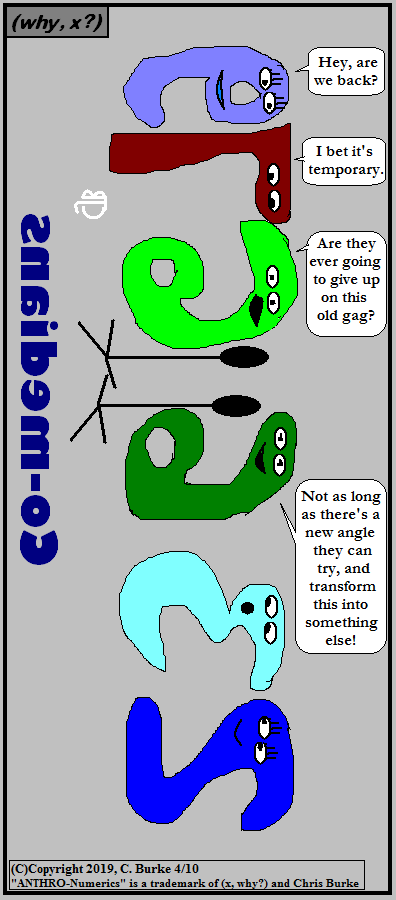Why, x? Why? Maybe why = x?

What's old is new again. Right?

Come back often for more funny math and geeky comics.## Monday, April 08, 2019

### Lateral Move

(Click on the comic if you can't see the full image.)We're all just puppets for our corporate (or civic) masters!

Recently, while going through old bins, I found one that contained mostly phtots and frames that I remember being on my desk at my old job. Some of these landed on my dresser at home (along with a lot that was already there). Also in the bin was a program from Lunacon 2003, a science-fiction convention foremerly held in Rye, NY. I'm saying this to establish a date.

Also in this bin, from years before this comic was ever thought of, was the stick puppet in the comic. The only two differences being that the puppet was not wearing glasses (I had a tendency not to wear mine around the house) and my daughter Jennifer's name was printed on the popsicle stick. There was also a note with some art work with it, which if they had once been attached, no longer were. I couldn't tell you how old this puppet is.

When I found it again, after not seeing it for many years, I laughed at the color scheme, since it matched the comic. I didn't know how I'd use it -- and then last week's story line started.

I hope you found it as amusing as I did.

Come back often for more funny math and geeky comics.## Saturday, April 06, 2019

### Creative Limbo, Part 2

(Click on the comic if you can't see the full image.)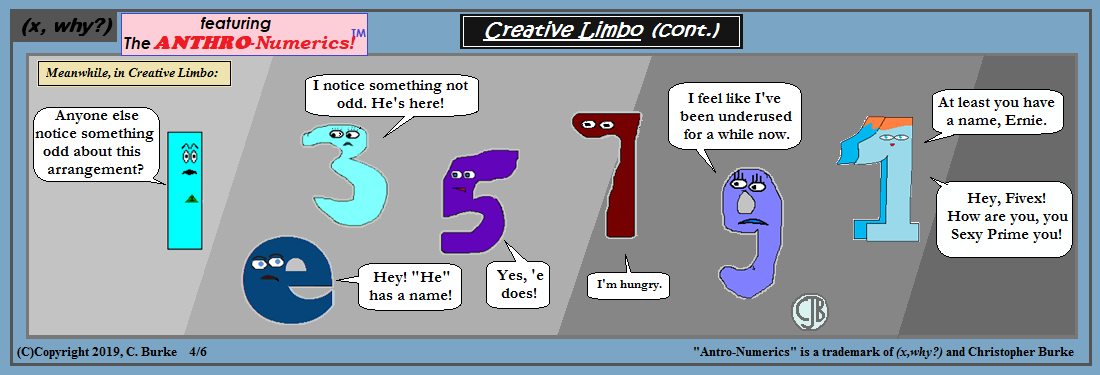The odd thing is how long it's been since I used Ernie!

I've used these Anthro-Numerics(tm) less over the past few years because they were mostly good for number puns. Plus, sometimes it's easier to create a new image if the joke is "out of character" for the existing number. But the strange thing is that I liked Ernie Nine (there's multiple jokes in there) but when I went to check, I haven't put him in a comic in over four years (with the exception of two comics with all the numbers and he's shrunken down a bit to fit the image.)

Speaking of shrinking down, I did that a bit in this comic just because I wanted to keep the same dimensions as yesterday's comic. Otherwise, I'd've gone with a bigger image.

Things will be back to normal ... sooner or later.
Thank you for indulging me.

The Anthrnumerics are a trademark of (x, why?) and Christopher Burke.

Come back often for more funny math and geeky comics.## Friday, April 05, 2019

### Creative Limbo

(Click on the comic if you can't see the full image.)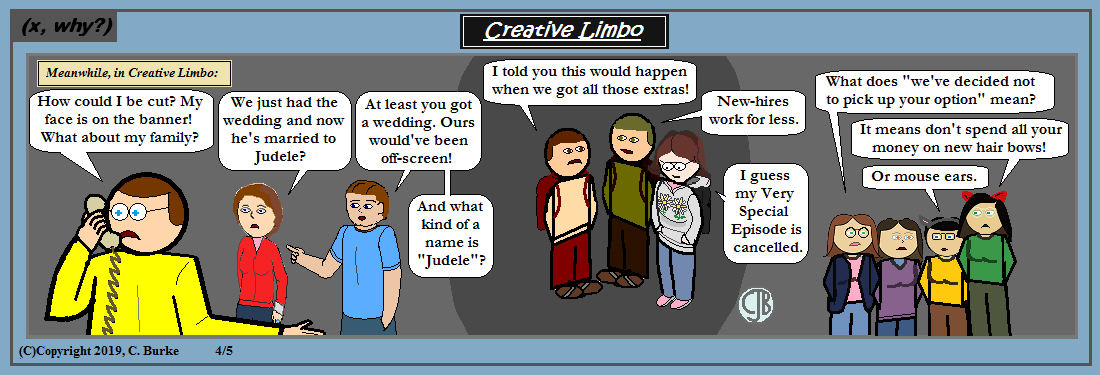New-hires work for less! Always a problem with you're paid according to a schedule.

Will the new girls be brought back? Will we ever see any Very Special Episodes? Time will tell!

Or you can tell me. Really, there's a place for comments on nearly every platform this is posted!

Come back often for more funny math and geeky comics.## Thursday, April 04, 2019

### Budget Cuts, Part 4

(Click on the comic if you can't see the full image.)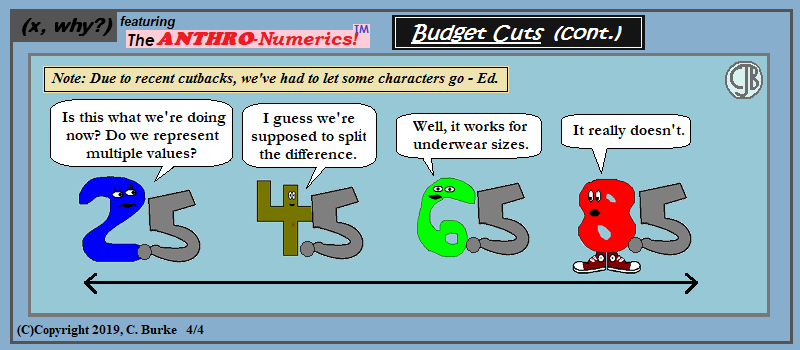Bad enough that odd-numbered waists above 33 are impossible to find with jeans, but underwear uses ranges like 34-36 and 38-40! What's a 37 to do? (Hopefully, I'll never find out, but the day may be coming!)

Come back often for more funny math and geeky comics.## Wednesday, April 03, 2019

### Budget Cuts, Part 3

(Click on the comic if you can't see the full image.)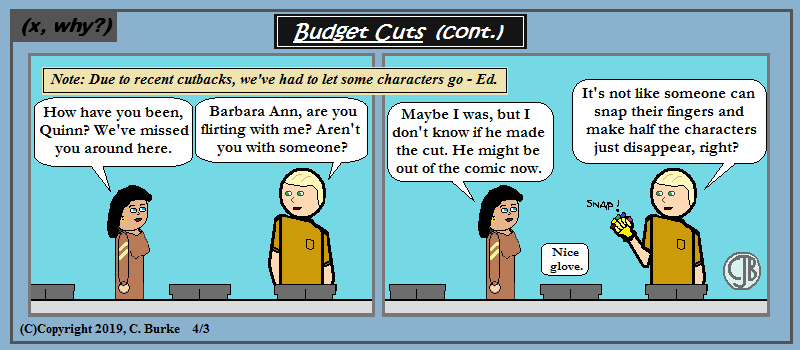I mean, really, who could have even think of such a thing? Not even space lizards who take over human hosts and pretend to be Egyptian gods by using weird alien tech!

Come back often for more funny math and geeky comics.## Tuesday, April 02, 2019

### Budget Cuts, Part 2

(Click on the comic if you can't see the full image.)Additionally, there may be some re-using of old story lines, or just wholesale borrowing of other stories. Or both.

Come back often for more funny math and geeky comics.## Monday, April 01, 2019

### Budget Cuts

(Click on the comic if you can't see the full image.)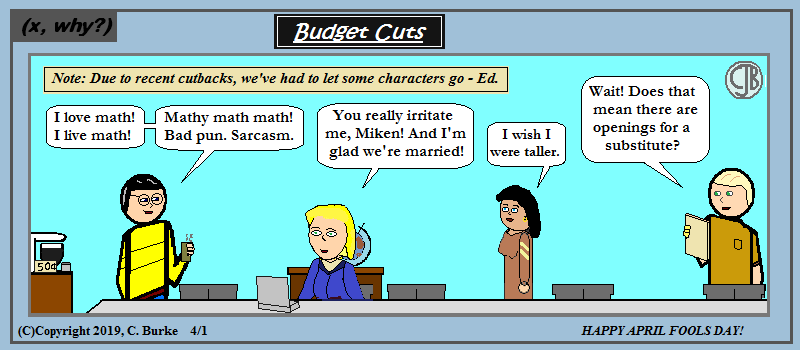Some substitution may occur, along with amalgamation.

Come back often for more funny math and geeky comics.